# CIE A Level Maths: Mechanics复习笔记4.1.2 Energy

### Kinetic Energy

There are many different forms of energy including, but not limited to, heat energy, light energy, chemical energy and nuclear energy. In this course we deal with a type of energy called mechanical energy which exists in two forms, kinetic energy (KE) and gravitational potential energy (GPE).

#### What is kinetic energy?

• Kinetic Energy is the energy formed by an object’s movement.
• Kinetic energy is a scalar quantity, it can not be negative
• The work done by a resultant force that acts to move an object in a particular direction will be equal to the change in kinetic energy of the object

#### How is kinetic energy calculated?

• A particle can only have kinetic energy when it is moving
• If a particle with mass, m kg is moving with speed v m s-1 then its kinetic energy can be calculated using the formula• If the particle is moving in two dimensions with the velocity vector then kinetic energy can be calculated in two ways
• Using the formula on each component individually and finding the sum of the KE in each component
• Finding the magnitude of the velocity to get the speed and then using the formula for KE
• Kinetic energy is measured in joules (J)
• 1 Kilojoule = 1000 joules (1 kJ = 1000 J)

#### How do we derive the formula for the change in kinetic energy?

• The work done by the resultant force is equal to the change in kinetic energy
• This is the final kinetic energy minus the initial kinetic energy
• The formula for the change in kinetic energy is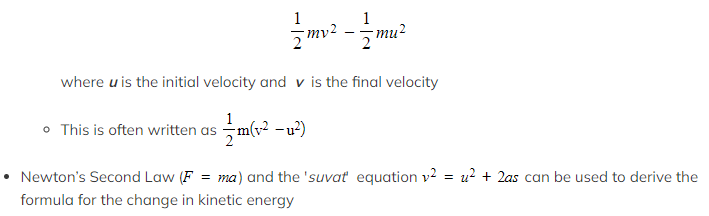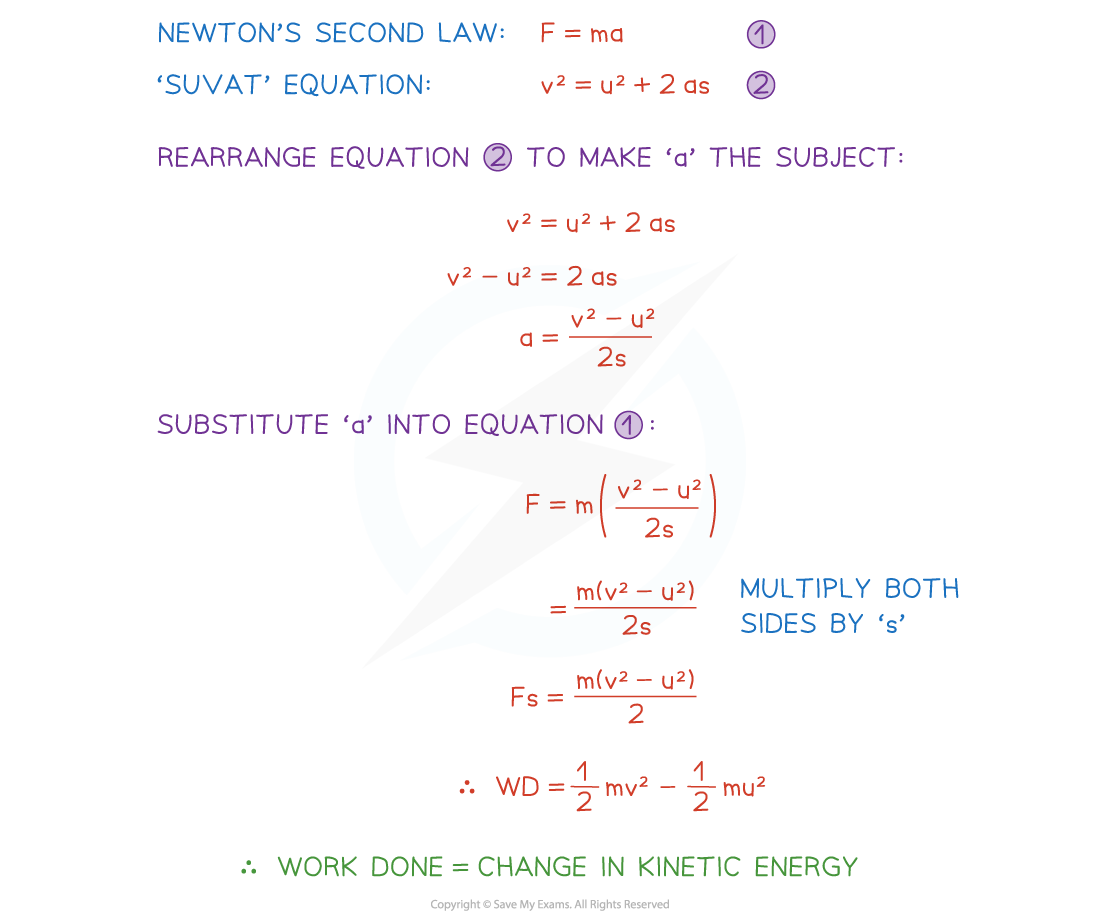#### Worked Example

A jogger increases her speed from 2 m s-1 to 3 m s-1 and her change in kinetic energy is 150 J, find the mass of the jogger.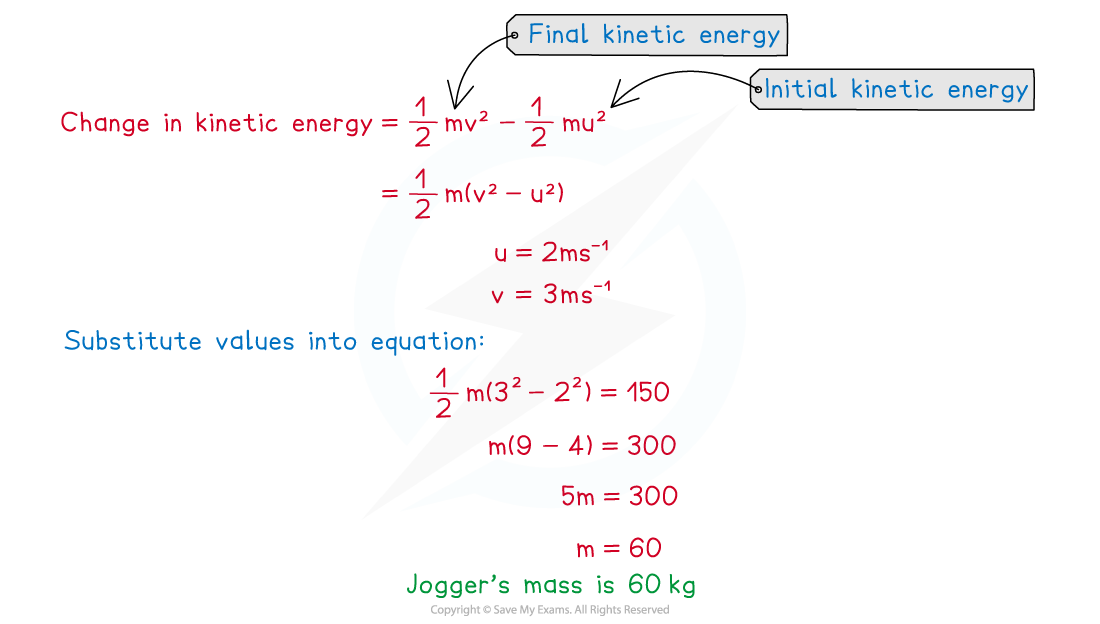#### Exam Tip

• Always double check the units are in kg for mass and m s-1 for velocity before carrying out any calculations.
• Be careful not to make the mistake of using the difference between the velocities with the equation, remember it should be the difference between the squares of the speeds.
• Make sure you are familiar with the method for the derivation of the formula for the change in kinetic energy.

### Gravitational Potential Energy

#### What is potential energy?

• Potential energy is the energy stored in a stationary object
• Gravitational potential energy (GPE) is the energy a particle possesses when it is at a fixed height and gravity is acting on it
• There are other types of potential energy but in this module only GPE is dealt with and so sometimes GPE is referred to as potential energy (PE)
• GPE will change as the vertical height of an object changes
• The work done against gravity on a particle as it moves upwards is equal to its increase in GPE
• The work done by gravity on a particle as it moves downwards is equal to its decrease in GPE

#### How is gravitational potential energy calculated?

• Gravitational potential energy is equal to the product of the force of the weight of an object and its vertical height, h , above a fixed point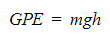• If the object is sitting on the ground or the point chosen as the fixed base level, the object will have no gravitational potential energy
• As the object moves upwards, its GPE will increase
• As the object moves downwards again, its GPE will decrease
• When mass is measured in kgacceleration due to gravity is measured in m s-2 and height is in metres, m, gravitational potential energy is measured in joules (J)
• 1 kilojoule = 1000 joules (1 kJ = 1000 J)

#### Worked Example

A ball of mass 400 grams is thrown vertically upwards from a height of 1 metre above the ground.  It reaches a maximum height of 4 metres before falling to the ground again. Stating clearly whether is represents a gain or a loss, write down the change in the gravitational potential energy of the ball

(i)between the instant it is thrown and the instant it reaches its maximum  height,
(ii)between the instant it is thrown and the instant it hits the ground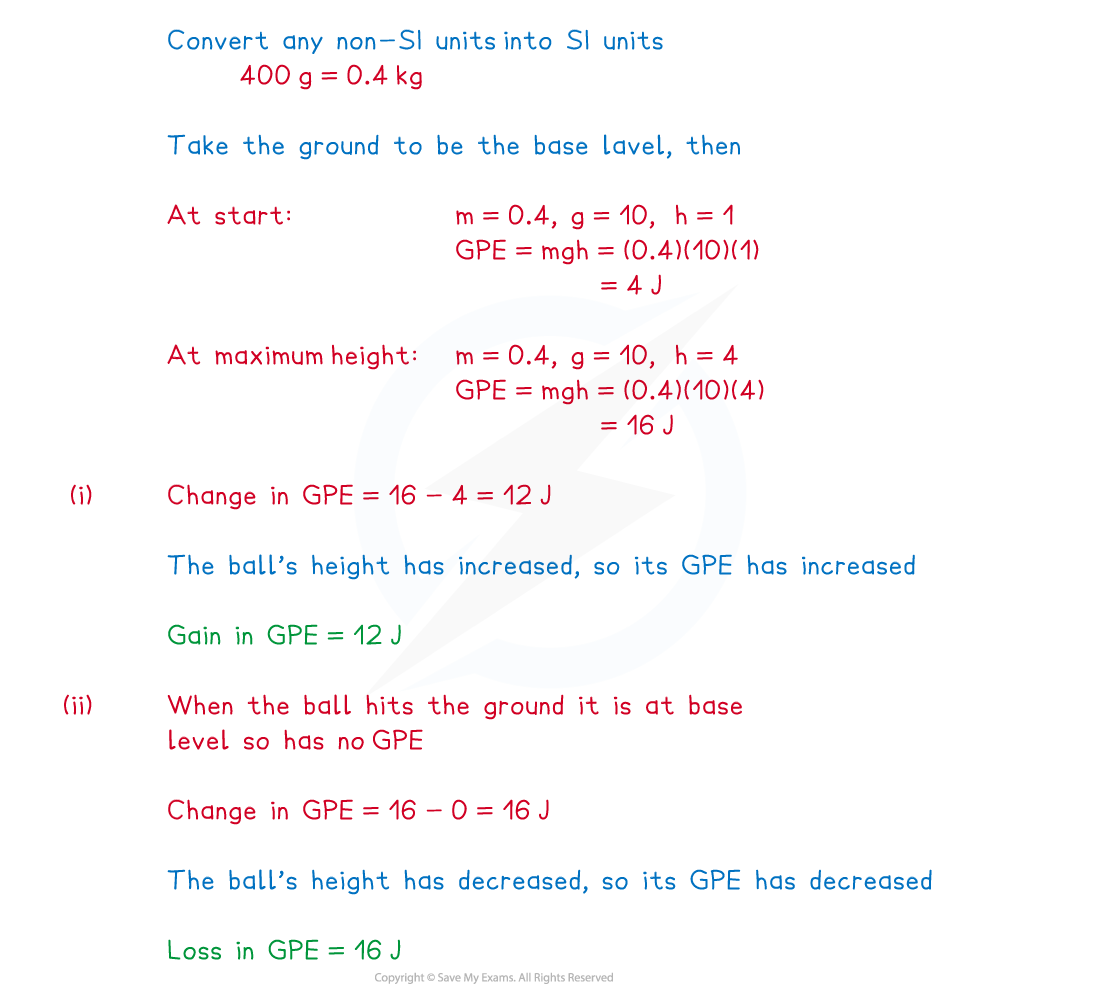#### Exam Tip

• Always double check the units are in kg for mass and m for height before carrying out any calculations.
• Remember that it is the vertical height that must be used within the calculations for GPE, if you are given the slant height you must use trigonometry to find the vertical height first.